# Multiplication principle + intersection of sets - math problems

#### Number of problems found: 8

• An aircraft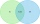An aircraft manufacturing company has submitted bids on two separate airline contracts, A and B. The company feels that it has a 70% chance of winning contract A and a 25% chance of winning contract B. Furthermore, it believes that winning contract A is i
• Hazard game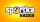In the Sportka hazard game, 6 numbers out of 49 are drawn. What is the probability that we will win: a) second prize (we guess 5 numbers correctly) b) the third prize (we guess 4 numbers correctly)?
• The box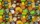The box contains five chocolate, three fruit, and two menthol candies. We choose sweets at random from the box. What probability will we take out one chocolate, one fruit, and one menthol candy without a return?
• Fall sum or sameFind the probability that if you roll two dice, it will fall the sum of 10, or the same number will fall on both dice.
• The universityAt a certain university, 25% of students are in the business faculty. Of the students in the business faculty, 66% are males. However, only 52% of all students at the university are male. a. What is the probability that a student selected at random in the
• Round destiny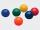There are 5 white and 10 red balls in the destiny. 4 balls will be drawn at random. What is the probability of the event "at least 2 spheres are white"?
• Dices throwsWhat is the probability that the two throws of the dice: a) Six falls even once b) Six will fall at least once
• Chambers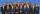The decision-making committee consists of three people. In order for the commission's decision to be valid, at least two members must vote in the same way. It is not possible not to vote in the commission, everyone only votes yes or no. We assume that the

We apologize, but in this category are not a lot of examples.
Do you have an exciting math question or word problem that you can't solve? Ask a question or post a math problem, and we can try to solve it.

We will send a solution to your e-mail address. Solved examples are also published here. Please enter the e-mail correctly and check whether you don't have a full mailbox.

Multiplication principle - math problems. Intersection of sets - math problems.